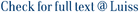The detection of patterns in multivariate time series is a relevant task, especially for large datasets. In this paper, four clustering models for multivariate time series are proposed, with the following characteristics. First, the Partitioning Around Medoids (PAM) framework is considered. Among the different approaches to the clustering of multivariate time series, the observation-based is adopted. To cope with the complexity of the features of each multivariate time series and the associated assignment uncertainty a fuzzy clustering approach is adopted. Finally, to neutralize the effect of possible outliers, a robust metric approach is used, i.e., the exponential transformation of dissimilarity measures. The proposed models are robust extensions of the Fuzzy C-Medoids clustering algorithm for multivariate time series. With respect to the management of the time behaviour, four variants are proposed: the Cross-Sectional Fuzzy C-Medoids clustering model with exponential transformation (CS-Exp-FCMd) classifies the multivariate time series taking into account their respective instantaneous features; the Longitudinal Fuzzy C-Medoids clustering model with exponential transformation (L-Exp-FCMd) takes into account the evolutive (longitudinal) features; the Mixed Fuzzy C-Medoids clustering model with exponential transformation (M-Exp-FCMd) which consider simultaneously both the instantaneous and the longitudinal features in the clustering process; the Dynamic Time Warping-based Fuzzy C-Medoids model with exponential transformation (DTW-Exp-FCMd) uses the Dynamic Time Warping (DTW) distance. Three simulation studies show the clustering performance of the proposed models in presence of outliers, compared to their non-robust counterparts, and to other models proposed in the literature. An application on real-world data on the concentration of three pollutants in nineteen stations in the Metropolitan City of Rome shows the relevance of the robustness to outliers in the identification of the clusters.

Robust fuzzy clustering of multivariate time trajectories / D'Urso, Pierpaolo; De Giovanni, Livia; Massari, Riccardo. - In: INTERNATIONAL JOURNAL OF APPROXIMATE REASONING. - ISSN 0888-613X. - 99:(2018), pp. 12-38. [https://doi.org/10.1016/j.ijar.2018.05.002]

### Robust fuzzy clustering of multivariate time trajectories

#### Abstract

The detection of patterns in multivariate time series is a relevant task, especially for large datasets. In this paper, four clustering models for multivariate time series are proposed, with the following characteristics. First, the Partitioning Around Medoids (PAM) framework is considered. Among the different approaches to the clustering of multivariate time series, the observation-based is adopted. To cope with the complexity of the features of each multivariate time series and the associated assignment uncertainty a fuzzy clustering approach is adopted. Finally, to neutralize the effect of possible outliers, a robust metric approach is used, i.e., the exponential transformation of dissimilarity measures. The proposed models are robust extensions of the Fuzzy C-Medoids clustering algorithm for multivariate time series. With respect to the management of the time behaviour, four variants are proposed: the Cross-Sectional Fuzzy C-Medoids clustering model with exponential transformation (CS-Exp-FCMd) classifies the multivariate time series taking into account their respective instantaneous features; the Longitudinal Fuzzy C-Medoids clustering model with exponential transformation (L-Exp-FCMd) takes into account the evolutive (longitudinal) features; the Mixed Fuzzy C-Medoids clustering model with exponential transformation (M-Exp-FCMd) which consider simultaneously both the instantaneous and the longitudinal features in the clustering process; the Dynamic Time Warping-based Fuzzy C-Medoids model with exponential transformation (DTW-Exp-FCMd) uses the Dynamic Time Warping (DTW) distance. Three simulation studies show the clustering performance of the proposed models in presence of outliers, compared to their non-robust counterparts, and to other models proposed in the literature. An application on real-world data on the concentration of three pollutants in nineteen stations in the Metropolitan City of Rome shows the relevance of the robustness to outliers in the identification of the clusters.
##### Scheda breve Scheda completa Scheda completa (DC)2018
Outlier time trajectory Cross-sectional and longitudinal clustering Dynamic time warping Exponential distance Robust fuzzy clustering Partitioning around medoids
Robust fuzzy clustering of multivariate time trajectories / D'Urso, Pierpaolo; De Giovanni, Livia; Massari, Riccardo. - In: INTERNATIONAL JOURNAL OF APPROXIMATE REASONING. - ISSN 0888-613X. - 99:(2018), pp. 12-38. [https://doi.org/10.1016/j.ijar.2018.05.002]
File in questo prodotto:
File
1-s2.0-S0888613X17306977-main.pdf

Solo gestori archivio

Descrizione: Articolo originale
Tipologia: Versione dell'editore
Licenza: Tutti i diritti riservati
Dimensione 4.65 MB
Utilizza questo identificativo per citare o creare un link a questo documento: `https://hdl.handle.net/11385/180629`
•42
•36